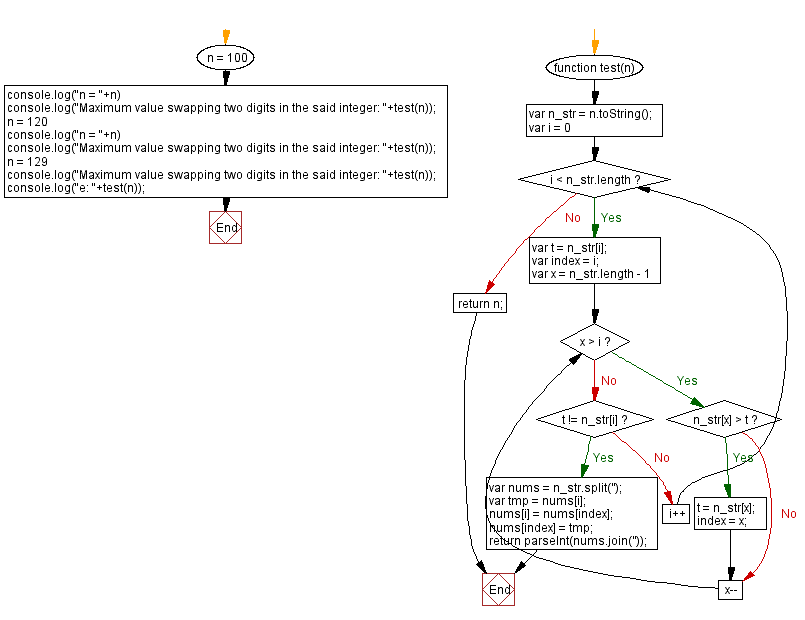# JavaScript: Maximum value swapping two digits in an integer

## JavaScript Math: Exercise-112 with Solution

Write a JavaScript program to calculate the maximum value by swapping two digits in a given integer.

Test Data:
(100) -> 100
(120) -> 210
(129) -> 921

Sample Solution:

HTML Code:

``````<!DOCTYPE html>
<html>
<meta charset="utf-8">
<title>JavaScript program to Maximum value swapping two digits in an integer</title>
<body>

</body>
</html>
```
```

JavaScript Code:

``````function test(n) {
var n_str = n.toString();

for (var i = 0; i < n_str.length; i++) {
var t = n_str[i];
var index = i;

for (var x = n_str.length - 1; x > i; x--) {
if (n_str[x] > t) {
t = n_str[x];
index = x;
}
}

if (t != n_str[i]) {
var nums = n_str.split('');

var tmp = nums[i];

nums[i] = nums[index];
nums[index] = tmp;

return parseInt(nums.join(''));
}
}
return n;
}

n = 100
console.log("n = "+n)
console.log("Maximum value swapping two digits in the said integer: "+test(n));
n = 120
console.log("n = "+n)
console.log("Maximum value swapping two digits in the said integer: "+test(n));
n = 129
console.log("Maximum value swapping two digits in the said integer: "+test(n));
console.log("e: "+test(n));
```
```

Sample Output:

```n = 100
Maximum value swapping two digits in the said integer: 100
n = 120
Maximum value swapping two digits in the said integer: 210
Maximum value swapping two digits in the said integer: 921
e: 921
```

Flowchart:Live Demo:

See the Pen javascript-math-exercise-112 by w3resource (@w3resource) on CodePen.

Improve this sample solution and post your code through Disqus

What is the difficulty level of this exercise?

Test your Programming skills with w3resource's quiz.

﻿